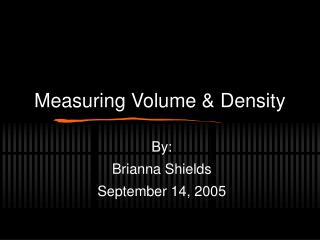DownloadDownload PresentationMeasuring Volume & Density

# Measuring Volume & Density

Télécharger la présentation## Measuring Volume & Density

- - - - - - - - - - - - - - - - - - - - - - - - - - - E N D - - - - - - - - - - - - - - - - - - - - - - - - - - -
##### Presentation Transcript

1. Measuring Volume & Density By: Brianna Shields September 14, 2005

2. DO NOW • 1. Name the unit used to label mass • 2. What is the first step for using a triple beam balance? • 3. What tool is used to measure length?

3. Definition The amount of space a liquid takes up Volume of a liquid

4. Tool Graduated Cylinder Volume of a liquid

5. Units Milliliters (ml) Volume of a liquid

6. Formula Read the volume level from the bottom of the meniscus Volume of a liquid

7. Definition The amount of space a solid takes up Volume of an irregular solid

8. Tool Graduated cylinder Volume of an irregular solid

9. Units Milliliter (ml) Volume of an irregular solid

10. Formula Measure the difference in water levels Volume of an irregular solid

11. Definition The amount of space a cube takes up Volume of a cube

12. Tool Metric Ruler Volume of a cube

13. Units Centimeters cubed (cm3) Volume of a cube

14. Formula Volume = length x width x height Volume of a cube

15. Example • What is the volume of the liquid at left?

16. Example • What is the volume of the liquid at left?

17. What is the volume of the object ?

18. Example • What is the volume of the cube at left?

19. Definition The number of particles that can be packed into a space Density

20. Tool Graduated cylinder or metric ruler AND triple beam balance Density

21. Units G.C. = g/ml Ruler = g/cm3 Density

22. Formula Density = mass divided by volume D =m/v Density v= l x w x h

23. How to calculate density 1. Find the mass in g 2. Find the volume in ml or cm3 3. Divide the mass by the volume 4. Round to the nearest tenth 5. Label answer with correct units Density

24. Water’s Density 1.0 g/ml Density of Water

25. Sink or Float? When d > 1.0 g/ml = sink When d< 1.0 g/ml = float Density

26. Estimating Density of sinking object is simply > 1.0 Density of floating object = % of object below water level Density

27. Cutting an object Density of a pure substance is always the same regardless of size Density

28. Example • What is the density of the object to the left if m=79.4 g, v= 29.8 cm3?

29. Which of the objects would sink? Float? Explain Densities of Common Elements and Compounds Water = 1.0 g/ml Methanol= .79 g/ml Gold 19.30 g/ml Air= .0013 g/ml Iron= 7.8 g/ml Ice= .93 g/ml Mercury= 13.5 g/ml Aluminum= 2.7 g/ml Ethanol= .94 g/ml Oak= 0.85 g/ml Example

30. How would these materials layer in a container? Densities of Common Elements and Compounds Water = 1.0 g/ml Methanol= .79 g/ml Gold 19.30 g/ml Air= .0013 g/ml Iron= 7.8 g/ml Ice= .93 g/ml Mercury= 13.5 g/ml Aluminum= 2.7 g/ml Ethanol= .94 g/ml Oak= 0.85 g/ml Example

31. Example • This block has a density of 10.0 g/cm3. What is the density of the block at the left if it is cut in half?

32. Practice • Work with your lab partner • Complete the volume and density practice questions in your handout • We will go over the correct answers next class

33. Hands On: Volume

34. Hands On: Volume

35. Hands On: Volume

36. Hands On: Volume

37. Hands On: Volume

38. Hands On: Density Object 1

39. Hands On: Density Object 1

40. Hands On: Density Object 2

41. Hands On: Density Object 2

42. Practice: Volume and Density

43. Practice: Volume and Density

44. Practice: Volume and Density

45. Practice: Volume and Density